NEET  >  Test: Electrical Energy & Power (NCERT)

# Test: Electrical Energy & Power (NCERT)

Test Description

## 5 Questions MCQ Test Physics Class 12 | Test: Electrical Energy & Power (NCERT)

Test: Electrical Energy & Power (NCERT) for NEET 2022 is part of Physics Class 12 preparation. The Test: Electrical Energy & Power (NCERT) questions and answers have been prepared according to the NEET exam syllabus.The Test: Electrical Energy & Power (NCERT) MCQs are made for NEET 2022 Exam. Find important definitions, questions, notes, meanings, examples, exercises, MCQs and online tests for Test: Electrical Energy & Power (NCERT) below.
Solutions of Test: Electrical Energy & Power (NCERT) questions in English are available as part of our Physics Class 12 for NEET & Test: Electrical Energy & Power (NCERT) solutions in Hindi for Physics Class 12 course. Download more important topics, notes, lectures and mock test series for NEET Exam by signing up for free. Attempt Test: Electrical Energy & Power (NCERT) | 5 questions in 5 minutes | Mock test for NEET preparation | Free important questions MCQ to study Physics Class 12 for NEET Exam | Download free PDF with solutions
 1 Crore+ students have signed up on EduRev. Have you?
Test: Electrical Energy & Power (NCERT) - Question 1

### If voltage across a bulb rated 220V, 100W drops by 2.5% of its rated value, the percentage of the rated value by which the power would decrease is

Detailed Solution for Test: Electrical Energy & Power (NCERT) - Question 1

Power, P = V2/R
As the resistance of the bulb is constant
∴ ΔP/P = 2ΔV / V
% decrease in power = ΔP/P × 100
= 2ΔV/V × 100
= 2 × 2.5% = 5%

Test: Electrical Energy & Power (NCERT) - Question 2

### Four wires of the same diameter are connected, in turn, between two points maintained at a constant potential difference. Their resistivities and lengths are; ρ and L (wire 1), 1.2ρ and 1.2L (wire 2), 0.9ρ and 0.9L (wire 3) and ρ and 1.5L (wire 4). Rank the wires according to the rates at which energy is dissipated as heat, greatest first,

Detailed Solution for Test: Electrical Energy & Power (NCERT) - Question 2

Resistance of a wire, R = ρl/A
Rate of energy dissipated as heat is
H = V2/R = V2A/ρl
For a wire 1,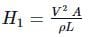For a wire 2,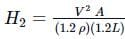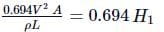For a wire 3,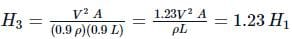For a wire 4,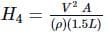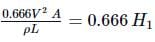∴H3 > H1 > H2 > H4.

Test: Electrical Energy & Power (NCERT) - Question 3

### A heater coil is rated 100 W, 200 V. It is cut into two idential parts. Both parts are connected together in parallel, to the same source of 200 V. The energy liberated per second in the new combination is

Detailed Solution for Test: Electrical Energy & Power (NCERT) - Question 3

The resistance of heater coil,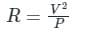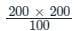= 400Ω
The resistance of either half part = 200 Ω.
Equivalent resistance when both parts are connected in parallel.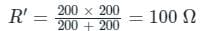The energy liberated per second when the combination is connected to a source of 200 V,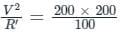= 400 J

Test: Electrical Energy & Power (NCERT) - Question 4

In the circuit shown in figure heat developed across 2Ω, 4Ω and 3Ωresistances are in the ratio

Detailed Solution for Test: Electrical Energy & Power (NCERT) - Question 4

Current through 2Ω,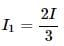Hence produced per second, H1
=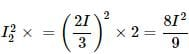Current through, 4Ω,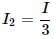Hence produced per second H2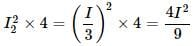Current through, 3Ω,I
Heat produced, H3 = I2 × 3 = 3I2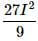∴ H1 : H2 : H3 = 8 : 4 : 27

Test: Electrical Energy & Power (NCERT) - Question 5

Two 2Ω resistances are connected in parallel in circuit X and in series in circuit Y. The batteries in the two circuits are identical and have zero internal resistance. Assume that the energy transferred to resistor A in circuit X within a certain time is W. The energy transferred to resistor B in circuit Y in the same time will be

Detailed Solution for Test: Electrical Energy & Power (NCERT) - Question 5

In a circuit X, both the resistance are in parallel, Therefore V is the same and, Power (energy transferred in unit time) is
V2/2 = W
In a circuit Y, both resistance are in series.
Therefore, VB + VB' = V or VB = V/2
In a circuit Y, power supplied to
B  =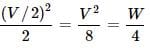## Physics Class 12

157 videos|425 docs|213 tests
 Use Code STAYHOME200 and get INR 200 additional OFF Use Coupon Code
Information about Test: Electrical Energy & Power (NCERT) Page
In this test you can find the Exam questions for Test: Electrical Energy & Power (NCERT) solved & explained in the simplest way possible. Besides giving Questions and answers for Test: Electrical Energy & Power (NCERT), EduRev gives you an ample number of Online tests for practice

## Physics Class 12

157 videos|425 docs|213 tests

### How to Prepare for NEET

Read our guide to prepare for NEET which is created by Toppers & the best Teachers